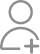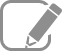Take Class 11 Tuition from the Best Tutors

•Affordable fees
•1-1 or Group class
•Flexible Timings
•Verified Tutors

Search in

# A die is thrown. Describe the following events:(i) A: a number less than 7 (ii) B: a number greater than 7 (iii) C: a multiple of 3(iv) D: a number less than 4 (v) E: an even number greater than 4 (vi) F: a number not less than 3Also find A ∪ B,A ∩ B,B ∪ C,E ∩ F,D ∩ E,A – C,D – E,,

Learn Exercise 16.2Follow 1AnswerWhen a die is thrown, the sample space is given by S = {1, 2, 3, 4, 5, 6}. Accordingly: (i) A = {1, 2, 3, 4, 5, 6} (ii) B = Φ (iii) C = {3, 6} (iv) D = {1, 2, 3} (v) E = {6} (vi) F = {3, 4, 5, 6} A ∪ B = {1, 2, 3, 4, 5, 6}, A ∩ B = Φ B ∪ C = {3, 6}, E ∩ F = {6} D ∩ E =Φ,...

When a die is thrown, the sample space is given by S = {1, 2, 3, 4, 5, 6}.

Accordingly:

(i) A = {1, 2, 3, 4, 5, 6}

(ii) B = Φ

(iii) C = {3, 6}

(iv) D = {1, 2, 3}

(v) E = {6}

(vi) F = {3, 4, 5, 6}

A ∪ B = {1, 2, 3, 4, 5, 6}, A ∩ B = Φ

B ∪ C = {3, 6}, E ∩ F = {6}

D ∩ E =Φ, A – C = {1, 2, 4, 5}

D – E = {1, 2, 3},Now ask question in any of the 1000+ Categories, and get Answers from Tutors and Trainers on UrbanPro.com

Find Class 11 Tuition near you

Looking for Class 11 Tuition ?

Learn from the Best Tutors on UrbanPro

Are you a Tutor or Training Institute?

Join UrbanPro Today to find students near you
X

### Looking for Class 11 Tuition Classes?

The best tutors for Class 11 Tuition Classes are on UrbanPro

• Select the best Tutor
• Book & Attend a Free Demo
• Pay and start Learning### Take Class 11 Tuition with the Best Tutors

The best Tutors for Class 11 Tuition Classes are on UrbanPro